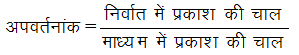# Physics

### Chapter : 4. Light Refraction

#### Refractive Index

Refractive Index
(a) Refractive Index in terms of Speed of Light:
The refractive index of a medium may be defined in terms of the speed of light as follows :OR(b) Refractive Index in terms of Wavelength :
Since the frequency () remains unchanged when light passes from one medium to another, therefore,(c) Relative Refractive Index :
The relative refractive index of medium 2 with respect to medium 1 is defined as the ratio of speed of light (V1) in the medium 1 to the speed of light (V2) in medium 2 and is denoted by 1µ2.-
Thus,As refractive index is the ratio of two similar physical quantities, so it has no unit and dimension. Factors On Which The Refractive Index Of A Medium Depends Are :
(i) Nature of the medium.
(ii) Wavelength of the light used.
(iii) Temperature
(iv) Nature of the surrounding medium.
It may be noted that refractive index is a characteristic of the pair of the media and also depends on the wavelength of light, but is independent of the angle of incidence.